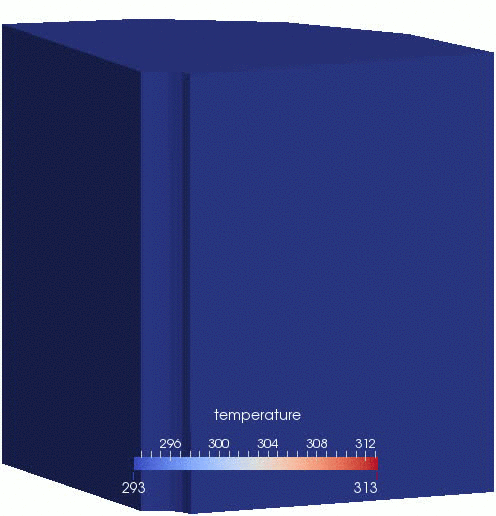# Porous Flow Tutorial Page 03. Adding heat advection and conduction

This Page adds heat conduction and advection with the fluid. The differential equation governing the temperature evolution is (1)

This equation is nonlinear since there are products of and , as well as the nonlinear function . Most of the nomenclature was used in Page 01, and the additional symbols introduced are:

• is time (units s)

• is the density of the rock grains (units kg.m)

• is the specific heat capacity of the rock grains (units J.kg.K)

• is the temperature (units K)

• is the specific heat capacity of the fluid (units J.kg.K)

• is the thermal conductivity of the rock-fluid system (units J.s.m.K). It is a tensor.

Before attempting to write an input file, a rough estimate of the expected nonlinear residuals must be performed, as discussed in convergence criteria. The residual for the Eq. (1) is approximately

(2)

where the parameters , , , , and , have been used in the final expression. In Page 02 the choice Pa.m was made. Choosing K.m yields

(3)

Note that this is significantly greater than the for the fluid equation. In the main, MOOSE can handle these types of discrepancies, but it is good practise to scale the variables so that their residuals are of similar magnitude. Therefore, a scaling factor of is applied to the temperature variable.

To model this thermo-hydro system, the PorousFlowBasicTHM action needs to be enhanced to read:

[Variables]
[./porepressure]
[../]
[./temperature]
initial_condition = 293
scaling = 1E-8
[../]
[]

[PorousFlowBasicTHM]
porepressure = porepressure
temperature = temperature
coupling_type = ThermoHydro
gravity = '0 0 0'
fp = the_simple_fluid
[]

(modules/porous_flow/examples/tutorial/03.i)

and some extra properties need to be added to the SimpleFluidProperties:

[Modules]
[./FluidProperties]
[./the_simple_fluid]
type = SimpleFluidProperties
bulk_modulus = 2E9
viscosity = 1.0E-3
density0 = 1000.0
thermal_expansion = 0.0002
cp = 4194
cv = 4186
porepressure_coefficient = 0
[../]
[../]
[]

(modules/porous_flow/examples/tutorial/03.i)

The boundary conditions used are the same as in Page 01 in addition to specifying a constant injection temperature of 313K:

  [./constant_injection_temperature]
type = PresetBC
variable = temperature
value = 313
boundary = injection_area
[../]

(modules/porous_flow/examples/tutorial/03.i)

Finally, some temperature-related Materials need to be defined

  [./thermal_expansion]
type = PorousFlowConstantThermalExpansionCoefficient
biot_coefficient = 0.8
drained_coefficient = 0.003
fluid_coefficient = 0.0002
[../]
[./rock_internal_energy]
type = PorousFlowMatrixInternalEnergy
density = 2500.0
specific_heat_capacity = 1200.0
[../]
[./thermal_conductivity]
type = PorousFlowThermalConductivityIdeal
dry_thermal_conductivity = '10 0 0  0 10 0  0 0 10'
block = 'caps aquifer'
[../]

(modules/porous_flow/examples/tutorial/03.i)

An animation of the results is shown in Figure 1. Readers are encouraged pause and explore the effect of changing parameters such as the rock thermal conductivity.Figure 1: Temperature evolution in the borehole-aquifer-caprock system.